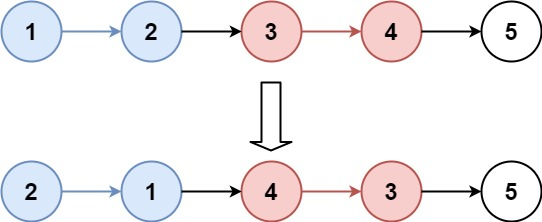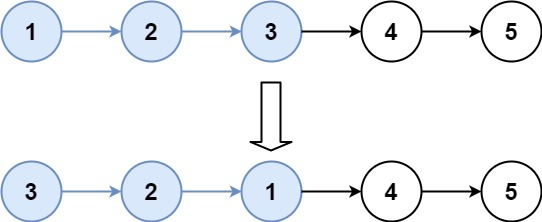GeetCode Hub

Given a linked list, reverse the nodes of a linked list k at a time and return its modified list.

k is a positive integer and is less than or equal to the length of the linked list. If the number of nodes is not a multiple of k then left-out nodes, in the end, should remain as it is.

You may not alter the values in the list's nodes, only nodes themselves may be changed.

Example 1:Input: head = [1,2,3,4,5], k = 2
Output: [2,1,4,3,5]

Example 2:Input: head = [1,2,3,4,5], k = 3
Output: [3,2,1,4,5]

Example 3:

Input: head = [1,2,3,4,5], k = 1
Output: [1,2,3,4,5]

Example 4:

Input: head = , k = 1
Output: 

Constraints:

• The number of nodes in the list is in the range sz.
• 1 <= sz <= 5000
• 0 <= Node.val <= 1000
• 1 <= k <= sz

Follow-up: Can you solve the problem in O(1) extra memory space?

/** * Definition for singly-linked list. * public class ListNode { * int val; * ListNode next; * ListNode() {} * ListNode(int val) { this.val = val; } * ListNode(int val, ListNode next) { this.val = val; this.next = next; } * } */ class Solution { public ListNode reverseKGroup(ListNode head, int k) { if(head == null){ return null; } if(k<=0){ return head; } ListNode dummy = new ListNode(0); dummy.next = head; ListNode curr = head; int count = 0; for(;count<k && curr!= null;count++){ curr = curr.next; } if(count == k){ ListNode prev = reverseKGroup(curr, k); for(int i=0;i<k;i++){ ListNode next = head.next; head.next = prev; prev = head; head = next; } return prev; } else{ return dummy.next; } } }

This is a tricky question, it requires knowledge of pointers, how we can reverse the node and call a recursive method with a new head pointer for subproblems. A detailed explanation is provided in the Video.

Time Complexity: O(n)

Space Complexity: O(n)